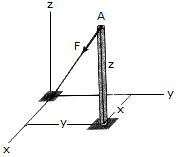# Engineering Mechanics - Force Vectors - Discussion

### Discussion :: Force Vectors - General Questions (Q.No.9)

9.The cable AO exerts a force on the top of the pole of F = {—120i — 90j — 80k} lb. If the cable has a length of 34 ft, determine the height z of the pole and the location (x,y) of its base.

 [A]. x = 16 ft, y = 16 ft, z = 25 ft [B]. x = 12 ft, y = 9 ft, z = 8 ft [C]. x = 20 ft, y = 10 ft, z = 14 ft [D]. x = 24 ft, y = 18 ft, z = 16 ft

Explanation:

No answer description available for this question.

 Sudipta Jana said: (Jul 11, 2014) Here the force f has its value along x, y, z directions are 120, 90, 80. So the resultant force is equal to 170. So the angles are along x, y, z cos inverse of (9120/170),(90/170),(80/170). Now the length of the cable is 34. So the components along, x axis 34*(120/170) = 24. y axis 34*(90/170) = 18. z axis 34*(80/170) = 16.

 Charles said: (Mar 21, 2018) How did you got the resultant force be 170?

 Grant said: (Sep 16, 2018) √ 120^2+90^2+80^2 = is the magnitude of the resultant force.